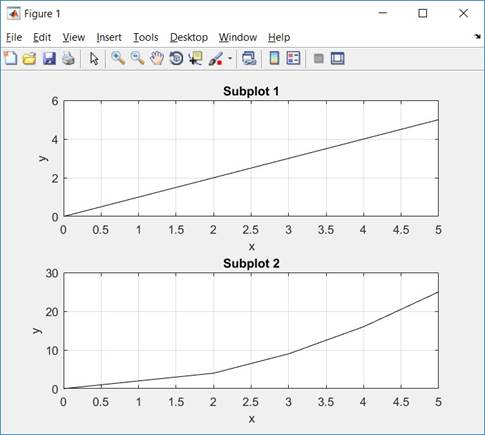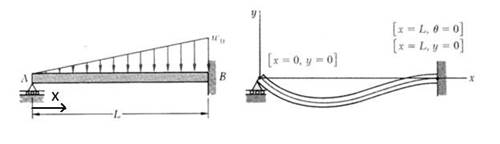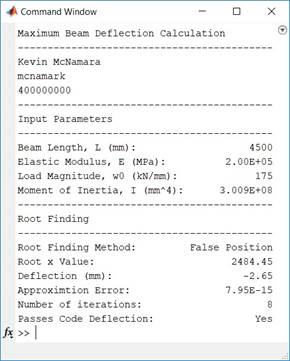# We Helped With This Engineering Homework: Have A Similar One?

SOLVEDCategory Engineering Other Undergraduate Solved Engineering Assignment Help

## Assignment Description

CivEng 2E03 Fall 2018 – Matlab Assignment 1

Matlab Review Materials

This document outlines some functions that will be helpful when you are working on this assignment. Subplots

In MATLAB, a figure is essentially a window containing a plot or plots (or other graphical items). We have created figures previously simply by using the plot() function. In these cases, all of our graphs have been on the sample plot within the figure. MATLAB has the capability to create multiple plots within a figure using the subplot() function:The subplot() function has the following syntax:

subplot(row,column,number) plot(x,y)

Where row tells MATLAB how many plots you want in the vertical direction, column tells how many in the horizontal direction, and number tells MATLAB which plot to work with. The subplot number goes from left to right, top to bottom. For example, to create a figure with 4 plots that are placed 2 by 2, the code is:

subplot(2,2,1) plot(x1,y1) subplot(2,2,2) plot(x2,y2) subplot(2,2,3) plot(x3,y3) subplot(2,2,4)

plot(x4,y4)

### Displaying to the Command Window using fprintf()

Until this point we usually have not worried about how to display output to the command window. However, it is often beneficial to be able to nicely format your output. The fprintf() function in MATLAB is key for creating formatted output. To simply output a string of text, the syntax is as follows:

### fprintf('Text to output. ')

The is necessary to tell MATLAB to go onto the next line. Without it, fprintf will continue to print in the same place. Try comparing the following:

to

### fprintf('Text to output. ')fprintf('Line 2 ')

Remember that MATLAB always encloses strings in single quotes ''. fprintf() is also great for nicely outputting values that were calculated within the code. This is achieved using a format string, and telling MATLAB which variables to output.

fprintf('format string ', variables)

For example, if you want to define a string of text outside of fprintf, and then display it:

### my_string = '2E03 is the best course.';fprintf('%s',my_string)

MATLAB interprets the %s as a string, and replaces its value with the string. This can be done with numbers and other values as well. For example:

this_year = 2017

fprintf('The Current Year is %d ',this_year)

this_year = 2017; this_day = 14; this_month = 'November';

fprintf('The current date is %s %d, %d. ',this_month, this_day, this_year)

%d is an integer value. To format a number with a certain number of decimal places, MATLAB uses %f:

x = 2.017859320;

### fprintf('The value of x to 2 decimal places is: %.2f ',x)

Where %.2f tells MATLAB to display two (.2) decimals.

For a more comprehensive review of fprintf(), take a look at the online MATLAB documentation, and test out the function’s capabilities.

## Assignment Description

Due: OCTOBER 24th, 2018 11:59pm

The purpose of this bonus lab is to consolidate your knowledge of the material on root finding methods and MATLAB functions from previous labs. As the new structural engineer working for a consulting firm, you’ve been asked to determine if the maximum deflection in the beam shown in Figure 1 exceeds the building code specified value of δallow = L/360. The beam is subjected to a linearly increasing distributed load, but your program should be flexible enough to allow for easy modification for other types of loading and support conditions.Figure 1 – Roller-fixed beam under linearly increasing distributed load

The equation for the deflection curve of the beam is given by:𝑤0                         5 +2𝐿2𝑥3−𝐿4𝑥)

𝑦(𝑥) =(−𝑥

120𝐸𝐼𝐿

You are given the following parameter values:

w0 = 175 kN/m (magnitude of the load) (note: the original instructions said kN/mm in error. If you already used this value you do not need to change it).

E =   200 000 MPa (modulus of elasticity)

I =    300.9x106 mm4 (second moment of area)

L =   length of the beam = the last non-zero digit of your student number (in m).                i.e. if student # is 400080006, L = 6 m                       if student # is 400082000, L = 2 m

You have been asked to write a set of MATLAB programs to solve this problem.

### Requirements (10 marks total)

1. (5 marks) Write a function to find the root of an equation. The root finding method you have to use depends on your lab session:

-       Monday – False Position

-       Thursday – Newton-Raphson

-       Friday – Secant

Your function should take the following inputs:

1)    Initial guess(es) – the number of initial guesses depends on what method you are using. Review your class notes if needed.

2)    f – an anonymous function you are seeking the root of.

3)    df – an anonymous function representing the derivative of the function you are seeking the root of (whether you need this depends on what method you are using).

Your function should return the following outputs:

1)    xr – the estimate of the root.

2)    eps_a – a vector containing the approximation error from each iteration – this will allow you to plot the error as a function of number of iterations.

3)    numit – the number of iterations required to reach the solution.

2. (3 marks) Write a script file that does the following (in this order):

1)    Define variables L = length of the beam, w0 = magnitude of load, I = moment of inertia, E = modulus of elasticity with the values given in this assignment.

2)    Define an anonymous function that computes the deflection, y(x), in the beam based on the given equation above. This function should take one input parameter, x, and should allow x to be a vector (i.e. use dot notation).

3)    Define a second anonymous function that computes the rotation, θ = dy/dx, based on the first function y(x). This function should take one input parameter, x, and should allow x to be a vector (i.e. use dot notation).

4)    Define a third anonymous function that computes the derivative of rotation = dθ/dx = d2y/dx2. This function should take one input parameter, x, and should allow x to be a vector (i.e. use dot notation). You may or may not need this depending on your root finding method.

5)    Plot a curve for deflection and rotation on two separate sets of axes (either on the same figure or two figures). Make sure that the graph has a title, labels on the axes, and turn on the grid. This will allow you to determine your initial guesses.

7)    Call your root finding function from Part 1 to find the location of maximum deflection within the beam.

8)    Plot the approximation error (eps_a) from your root finding against iteration number to show how the function converges as the number of iterations increases. Make sure that the graph has a title, labels on the axes, and turn on the grid.

9)    Check whether the maximum deflection of the beam is less than the code specified limit of L/360.

3. (2 marks) Create a summary output to the command window with the following information:

1)    Your name, macid, and student number (Abanoub Gaber, Gabera1, 400061946)

2)    Input parameters

3)    Root finding method used

4)    Root x value approximation (xr)

5)    Deflection at the root xr = y(xr)

6)    Final approximation error of the root (eps_a)

7)    Number of iterations required to reach approximation (numit)

8)    Whether or not the maximum deflection of the beam is less than the code specified limit of L/360.

Your output should look similar to this (note that the numbers below are not the same as the answer you should find).Ensure that there is a block of comments at the top of all files with the following information:

1)    A title of the script/function

2)    A short description of the file, listing the inputs and outputs

4)    Your macid and student number

### Submission Instructions

Submit BOTH your root finding function and the script file to the Avenue dropbox by the due date in a compressed ZIP folder. Name the folder “CE2E03_A1_MACID.zip”, replacing MACID with your Mac ID (i.e. CE2E03_A1_mcnamark.zip). General Hints and Tips

1)    Use the equation in your textbook to select an appropriate stopping criterion to an appropriate number of significant figures. For most civil engineering problems 5 significant figures are sufficient.

2)    Be sure to include a stopping criterion based on the number of iterations so that the function does not run indefinitely. If the program reaches the maximum number of iterations, make sure to print this out in some way so that the user knows that the result is not correct.

3)    The maximum deflection of a beam corresponds to the point where the rotation or slope (dy/dx) is zero.

Is it free to get my assignment evaluated?

Yes. No hidden fees. You pay for the solution only, and all the explanations about how to run it are included in the price. It takes up to 24 hours to get a quote from an expert. In some cases, we can help you faster if an expert is available, but you should always order in advance to avoid the risks. You can place a new order here.

How much does it cost?

The cost depends on many factors: how far away the deadline is, how hard/big the task is, if it is code only or a report, etc. We try to give rough estimates here, but it is just for orientation (in USD):

 Regular homework \$20 - \$150 Advanced homework \$100 - \$300 Group project or a report \$200 - \$500 Mid-term or final project \$200 - \$800 Live exam help \$100 - \$300 Full thesis \$1000 - \$3000

How do I pay?

Credit card or PayPal. You don't need to create/have a Payal account in order to pay by a credit card. Paypal offers you "buyer's protection" in case of any issues.

Why do I need to pay in advance?

We have no way to request money after we send you the solution. PayPal works as a middleman, which protects you in case of any disputes, so you should feel safe paying using PayPal.

Do you do essays?

No, unless it is a data analysis essay or report. This is because essays are very personal and it is easy to see when they are written by another person. This is not the case with math and programming.

Why there are no discounts?

It is because we don't want to lie - in such services no discount can be set in advance because we set the price knowing that there is a discount. For example, if we wanted to ask for \$100, we could tell that the price is \$200 and because you are special, we can do a 50% discount. It is the way all scam websites operate. We set honest prices instead, so there is no need for fake discounts.

Do you do live tutoring?

No, it is simply not how we operate. How often do you meet a great programmer who is also a great speaker? Rarely. It is why we encourage our experts to write down explanations instead of having a live call. It is often enough to get you started - analyzing and running the solutions is a big part of learning.

What happens if I am not satisfied with the solution?

Another expert will review the task, and if your claim is reasonable - we refund the payment and often block the freelancer from our platform. Because we are so harsh with our experts - the ones working with us are very trustworthy to deliver high-quality assignment solutions on time.

## Popular Solved Assignments Like This

Customer Feedback

"Thanks for explanations after the assignment was already completed... Emily is such a nice tutor! "

Order #13073